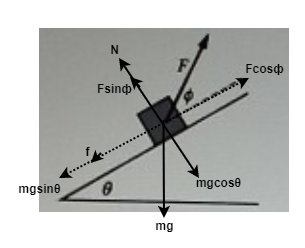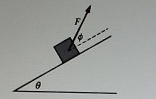# Problem: A box of mass m is on a rough inclined plane that is at an angle theta with the horizontal. A force of magnitude F at an angle ф with the plane is exerted on the block as shown above as the block moves up the plane there is a frictional force between the plane and magnitude F what is the magnitude of the net force acting on the box?a. F sinф  - mgcos θ - fb. F cos (ф+θ)  + mgsin θ - fc. F cosф  - mgsin θ - fd. F cos (ф+θ)  - mgsin θ - f

###### FREE Expert Solution

Let's consider the block's free-body diagram below:82% (125 ratings)###### Problem DetailsA box of mass m is on a rough inclined plane that is at an angle theta with the horizontal. A force of magnitude F at an angle ф with the plane is exerted on the block as shown above as the block moves up the plane there is a frictional force between the plane and magnitude F what is the magnitude of the net force acting on the box?

a. F sinф  - mgcos θ - f

b. F cos (ф+θ)  + mgsin θ - f

c. F cosф  - mgsin θ - f

d. F cos (ф+θ)  - mgsin θ - f

Frequently Asked Questions

What scientific concept do you need to know in order to solve this problem?

Our tutors have indicated that to solve this problem you will need to apply the Inclined Planes with Friction concept. You can view video lessons to learn Inclined Planes with Friction. Or if you need more Inclined Planes with Friction practice, you can also practice Inclined Planes with Friction practice problems.

What professor is this problem relevant for?

Based on our data, we think this problem is relevant for Professor Tsai's class at UCSD.